# How to delete only one row in csv with Python?

In this tutorial, we will learn to delete only one row in csv with python. We will be using the Pandas library. Pandas is an open-source library for data analysis; it is one of the most popular python libraries to investigate the data and insights. It includes several functionalities to perform operations on data sets. It can be combined with other libraries like NumPy to perform specific functions with the data.

We will use the drop() method to delete the row from any csv file. In this tutorial, we will illustrate three examples to delete the row from the csv file using the same method. By the end of this tutorial, you will be familiar with the concept and able to delete the row from any csv file.

## Syntax

This is the syntax to delete multiple rows from an array.

#drop method
data = data.drop(data.index[index_number])
print(data)


In this syntax, we first read the data frame; then we use the method to pass through the index and delete them. Finally, we printed the updated data.

## Example 1: Delete Last Row from the Csv File

Here’s an example, where we deleted the last row using drop method. First, we read the CSV file as a Data Frame using read_csv(), then used the drop() method to delete the row at index -1. We then specified the index to drop using the index parameter. Finally, we write the updated DataFrame back to the CSV file using to_csv(), setting index=False to avoid writing the row indices to the file.

import pandas as pd
df = df.drop(df.index[-1])
df.to_csv('How_to_delete_only_one_row_in_CSV_with_Python.csv', index=False)


## Output

CSV file before running code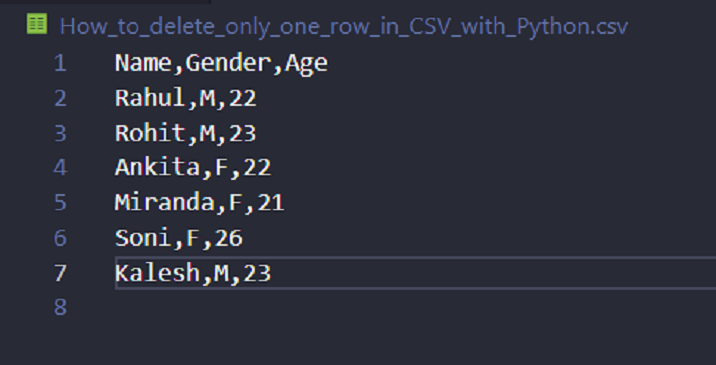CSV file after running code −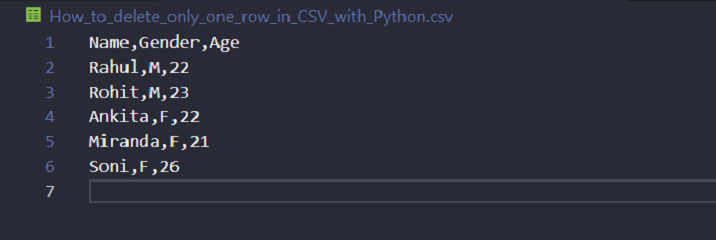## Example 2: Delete a Row by Label

Here’s a similar example as above; in this example we are deleting the row with the label ‘row’. In this example, we read in the CSV file using read_csv(), but this time we set the 'id' column as the index using the index_m parameter. Then, we use the drop() method to delete the row with the index label ‘row’. We then specified the label to drop using the index parameter. Finally, we write the updated DataFrame back to the CSV file using to_csv(), without setting index=False because the row labels are now part of the CSV file.

import pandas as pd
df = df.drop('row1')
df.to_csv('How_to_delete_only_one_row_in_CSV_with_Python.csv', index=True)


## Output

CSV file before running code −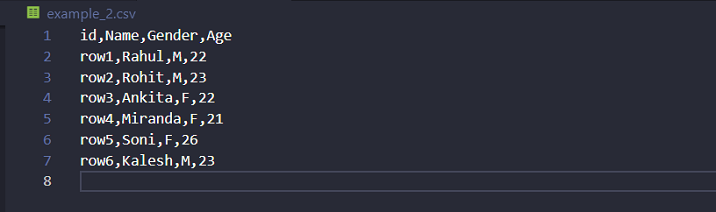CSV file after running code −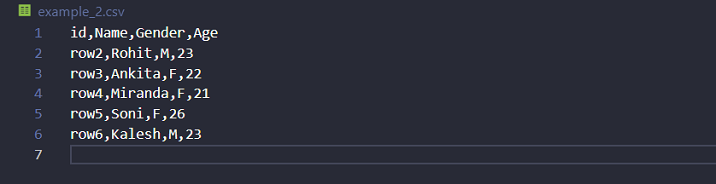## Example 3: Delete a Row with Condition

In this example, first, we read the CSV file, then use the drop() method to delete the row where the value in the 'Name' column is equal to ‘John’. We do this by first using Boolean indexing to select the rows that meet the condition. Finally, we write the updated DataFrame back to the CSV file using to_csv(), again setting index=False.

import pandas as pd
df = df.drop(df[df.Name == 'Ankita'].index)
df.to_csv('example_3.csv', index=False)


## Output

CSV file before running code −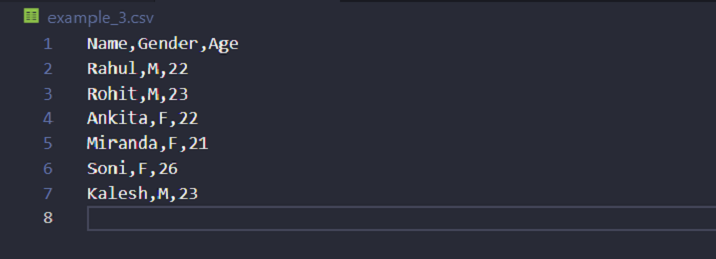CSV file after running code −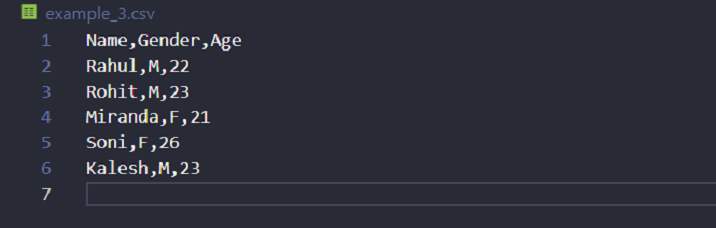## Conclusion

We learned that pandas is a powerful and flexible Python library for data manipulation and analysis. It provides high-performance data structures. We illustrated the drop method to delete the row from the csv file. Depending on needs, we can specify the rows to drop by index, label, or condition. This method allows to delete one or more rows from the csv file.

Updated on: 12-May-2023

6K+ Views